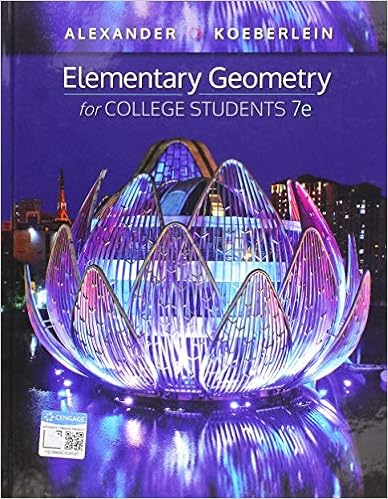# Programming exercises 1 in figure 3 10 the process of

• 981
• 70% (23) 16 out of 23 people found this document helpful

This preview shows page 341 - 347 out of 981 pages.

##### We have textbook solutions for you!
The document you are viewing contains questions related to this textbook.The document you are viewing contains questions related to this textbook.
Chapter PC / Exercise 10
Elementary Geometry for College Students
Alexander/KoeberleinExpert Verified
Programming Exercises 1. In Figure 3-10 the process of buying and planting flowers in the spring was sh Answer : Student answers will vary widely. They should come up with processes that fit th if it’s time for your annual teeth cleaning then call the dentist for an appointment while the day and time you want isn’t available if another time the same day is available make the appointment at the new time on else pick a new day and time endif endwhile write appointment on your calendar endif if you want to take a class this semester then try to register for the class while the class is full if another section is available enroll for the available section else select a new class endif endwhile print schedule endif 2. Each of the flowchart segments in Figure 3-24 is unstructured. Redraw each f Answer :
##### We have textbook solutions for you!
The document you are viewing contains questions related to this textbook.The document you are viewing contains questions related to this textbook.
Chapter PC / Exercise 10
Elementary Geometry for College Students
Alexander/KoeberleinExpert Verified
a. b. c. d. e. 3. Write pseudocode for each example (a through e) in Exercise 2 making sure y Answer : a. do A while B is true do C do A endwhile b. do D if E is true then do H do I else do F if G is true then do I endif endif c. do k
if L is true then do P while Q is true do P endwhile do R else do M do N if O is true then do R endif endif d. do S if T is true then do Y if Z is true then do V if W is true then do A else do X endif else do A endif else do U do V if W is true then do A else do X endif endif e. if B is true then do G while H is not true do I do G
endwhile do D while E is true do I do D endwhile do F else do C do D while E is true do I do D endwhile do F endif 4. Assume you have created a mechanical arm that can hold a pen. The arm can Lower the pen to a piece of paper. Raise the pen from the paper. Move the pen 1 inch along a straight line. (If the pen is lowered, this action draws a Turn 90 degrees to the right. Draw a circle that is 1 inch in diameter. Draw a structured flowchart or write structured pseudocode describing the logic t a. a 1-inch square b. a 2-inch by one-inch rectangle c. a string of three beads d. a short word (for example, cat ) e. a four-digit number Answer : This solution assumes the above tasks are labeled as follows: A. Lower the pen to a piece of paper. B. Raise the pen from the paper. C. Move the pen one inch along a straight line. (If the pen is lowered, D. Turn 90 degrees to the right. E. Draw a circle that is one inch in diameter. a. a one-inch square Pseudocode :
start lower the pen to a piece of paper move one inch along a straight line turn 90 degrees to the right move one inch along a straight line turn 90 degrees to the right move one inch along a straight line turn 90 degrees to the right move one inch along a straight line raise the pen from the paper stop or start do A do C do D do C do D do C do D do C do B stop Flowchart: b. a two-inch by one-inch rectangle do start
Pseudocode : start lower the pen to a piece of paper move one inch along a straight line move one inch along a straight line turn 90 degrees to the right move one inch along a straight line turn 90 degrees to the right move one inch along a straight line move one inch along a straight line turn 90 degrees to the right move one inch along a straight line
•••